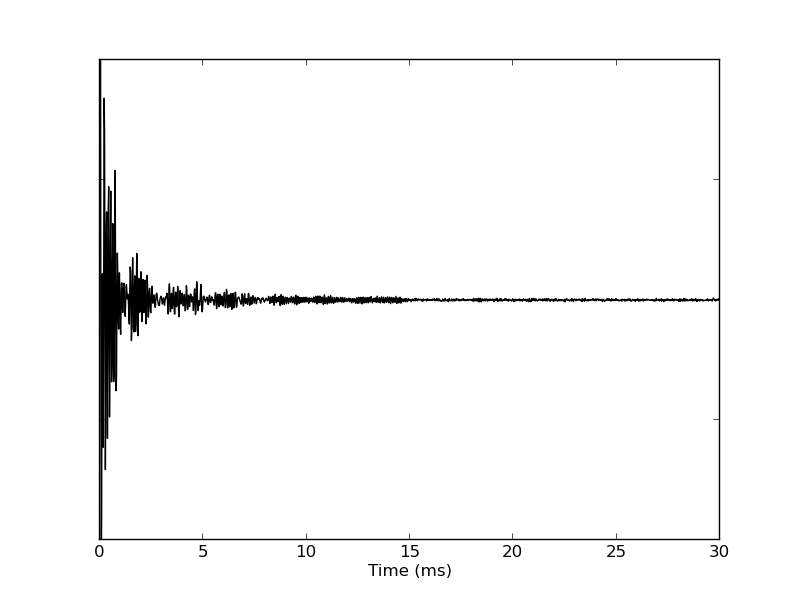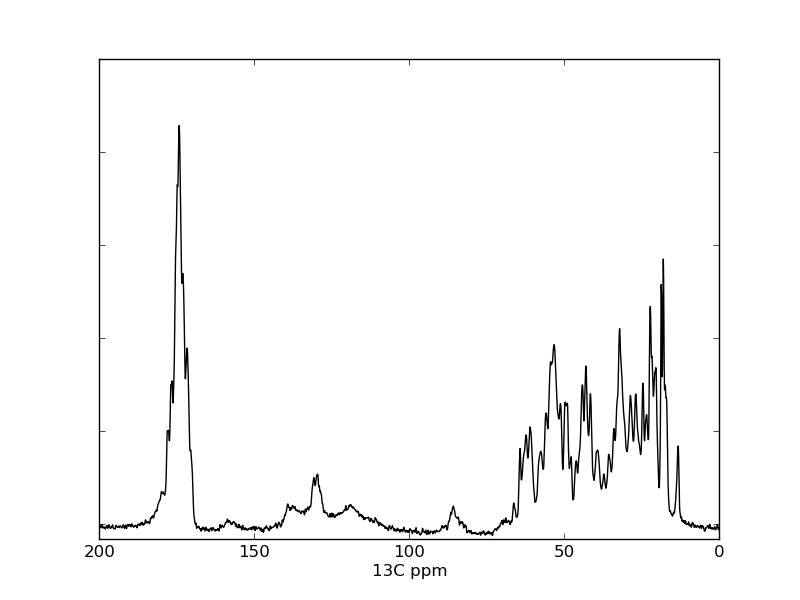# 1D visualization examples¶

## Introduction¶

These two examples are taken from Listing S2 and S3 in the 2013 JBNMR nmrglue paper. In the first example a the 1D time domain signal from a NMRPipe file (test.fid) is visualized using the plot_1d_pipe_time.py script. In the second example a portion of a 1D 13C CP MAS NMR spectrum is visualized from the NMRPipe file test.ft using the script plot_1d_pipe_freq.py

## Instructions¶

Execute python plot_1d_pipe_time.py to visualize the data in the file test.fid. The resulting file fid.png is presented as Figure 2 in the paper.

Execute python plot_1d_pipe_spectrum.py to visualize the data in the file test.ft. The resulting file spectrum.png is presented as Figure 3 in the paper.

Listing S2

import nmrglue as ng
import matplotlib.pyplot as plt

# read in the data from a NMRPipe file

# make a unit conversion object for the axis
uc = ng.pipe.make_uc(dic, data)

# plot the spectrum
fig = plt.figure()
ax.plot(uc.ms_scale(), data.real, 'k-')

# decorate axes
ax.set_yticklabels([])
ax.set_xlabel("Time (ms)")
ax.set_ylim(-100000, 100000)

# save the figure
fig.savefig("fid.png")Listing S3

import nmrglue as ng
import matplotlib.pyplot as plt

# read in the data from a NMRPipe file

# create a unit conversion object for the axis
uc = ng.pipe.make_uc(dic, data)

# plot the spectrum
fig = plt.figure()